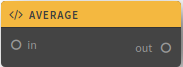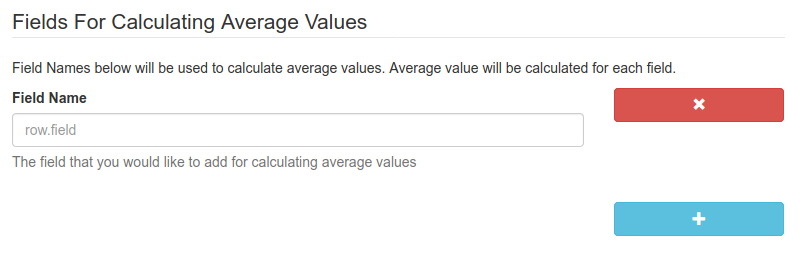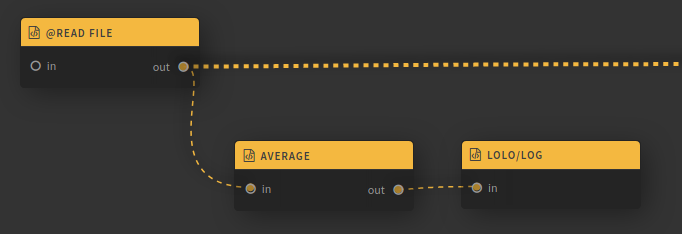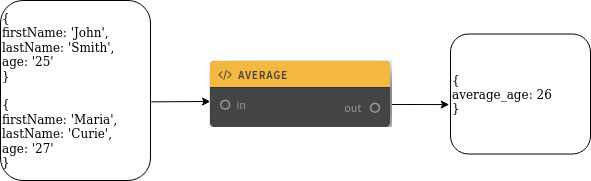# Overview

Calculates an average values of defined fields## Description

This function is used to calculate the arithmetic mean for all events. You can specify several fields at once for which you want to get the arithmetic mean. The result of the function is an object containing the fields 'average_' and the value.
The function does not change the content of the original event.

## Function Schema## Examples

Let's calculate the average age of all students. To do this, we send events containing data about students to the function input. Insert the function block parallel to the main event processing thread. In the function parameters, set Field Name= 'age'. At the output of the function, we get object
{ average_age: }